# Slew Rate: What is it? (Formula, Units & How To Measure It)

Contents

## What is Slew Rate?

In electronics, the slew rate is defined as the maximum rate of output voltage change per unit time. It is denoted by the letter S. The slew rate helps us to identify the amplitude and maximum input frequency suitable to an operational amplifier (OP amp) such that the output is not significantly distorted.

The slew rate should be as high as possible to ensure the maximum undistorted output voltage swing.

Slew rate is a critical factor in ensuring that an OP amp can deliver an output that is reliable to the input. Slew rate changes with the change in voltage gain. Therefore, it is generally specified at unity (+1) gain condition.

A typically general-purpose device may have a slew rate of 10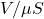. This means that when a large step input signal is applied to the input, the electronic device can provide an output of 10 volts in 1 microsecond.

## Slew Rate Formula

The equation for the slew rate is given by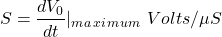Where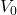is the output produced by the amplifier as a function of time t.

## Slew Rate Units

The slew rate of an electronic circuit is defined as the rate of change of the voltage per unit of time. The units for slew rate are Volts per second or.

## How to Measure Slew Rate?

The slew rate is measured by applying a step signal to the input stage of the op-amp and measuring the rate of change occurs at the output from 10% to 90% of the output signal’s amplitude. Generally, the applied step signal is large and it is about 1 V.

The slew rate is measured from the output voltage waveform as: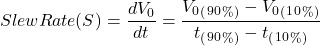The slew rate can be measured by using an oscilloscope and a function generator.

The circuit used for slew rate measurement is shown in the figure below.

The input and slew limited output voltage waveform are shown in the figure below.

## Slew Rate of OP Amp

Slew rate decides the capability of an op-amp to change its output rapidly, hence it decides   the highest frequency of the operation of a given op-amp.

The Slew rate of the op-amp can limit the performance of a circuit and it can distort the output waveform if its limit is exceeded.

Op-amps may have different slew rates for positive and negative transitions because of the circuit configuration.

The Slew rate should be ideally infinite and practically as high as possible. The Slew rate of IC 741 op-amp is only about 0.5which is its major drawback. Therefore, it cannot be used for high-frequency applications.

### Slew Rate Limiting in Amplifiers

#### High Gain at the Input Stage of OP-Amp:

The modern operational amplifiers use high gain differential input stages with trans-conductance characteristics. This means that an amplifier takes a differential input voltage at the input stage and produces an output current at the output stage. Note that trans-conductance is nothing but the transfer conductance, also called as mutual conductance, it is the electrical characteristic and is defined as the current through the output of a device to the voltage across the input of a device. Mathematically it is expressed as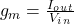.

This trans-conductance of amplifiers is typically very high, also at this point, the large open-loop gain of the amplifier is produced. This means that the small input voltage can saturate the input stage. In this saturation condition, the stage produces approximately constant output current and acts as a constant current source. In this condition, the rate of change occurs at the output of the amplifiers is severely limited. This limits the slew rate of an OP-amp.

#### Frequency Compensation at the Second Stage of OP-Amp:

To provide stability, frequency compensation is used in all op-amps to reduce the high-frequency response have a considerable effect on slew rate. A reduced frequency response limits the rate of change that occurs at the output of the amplifiers and hence it affects the slew rate of an op-amp.

Now, the frequency compensation at the second stage of the op-amp is the low pass characteristic and it is similar to an integrator. Hence constant current input will produce a linearly increasing output. If the second stage has an effective input capacitance C and voltage gain A2, then the slew rate can be expressed as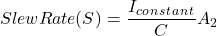Where Iconstant is the constant current of the first stage in saturation.

#### Temperature:

The slew rate is a temperature-dependent parameter. A positive slew rate occurs when a signal is rising and the negative slew rate occurs when a signal is falling. Typically, the slew rate of an amplifier will increase with increasing temperature.

## Slew Rate vs Bandwidth

### Slew Rate

Slew rate is the maximum rate at which an amplifier can respond to the sudden change of input level. Slew rate can distort (or limit) any signal amplified by an op-amp.

The sinusoidal input signal multiplied by the gain of the op-amp results in a slope which is higher than the slew rate of the op-amp. Hence output waveform will be a straight line instead of a curving section of the sinusoidal. This effect is non-linear. Thus, slewing can modify or distort the shape of a signal.

### Bandwidth

The Bandwidth or power bandwidth of an amplifier is the range of frequencies for which all the signal frequencies are amplified almost equally (without distortion) also poles in the transfer function of op-amp leads to low-pass-filter behavior i.e., the amplitude of the signal decreases as frequency increases, and phase shift occurs. This effect is linear and they do not produce distortion into the output signal.

The bandwidth of an op-amp should be as large as possible. It should be capable of amplifying the signal from zero frequency. Thus, the gain of an op-amp should be constant from 0 frequency to infinite frequency. Bandwidth is expressed in hertz.

### Relation Between Slew Rate and Full Power Bandwidth

Assuming that the input signal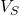is a sine wave, we can obtain the value of maximum frequency for which the amplifier produces an undistorted output.

(1)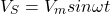Now, for a unity gain non-inverting amplifier, the output is exactly equal to the input.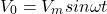Differentiate the above equation both the sides we get,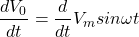(2)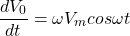Now,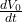will be maximum when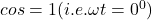and maximum value ofis nothing but the slew rate S. Put it into equation (2) we get,

(3)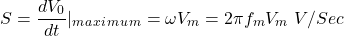Where,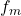= the maximum signal frequency in Hz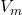= the maximum peak voltage of the signal

Rearranging the term in equation (3), we get

(4)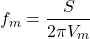The above equation indicates the highest frequency at which the peak-to-peak output voltage swing is equal to the DC output voltage range. In other words, it is the maximum frequencyfor which the amplifier produces an undistorted output. It is called as full power bandwidth. It is also sometimes described as the slew-rate-limited-bandwidth.

### Slew Rate Calculation

An operational amplifier is required to amplify a signal with a peak voltage of 5 volts at a frequency of 20kHz. Find out a slew rate.

Given data: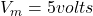,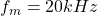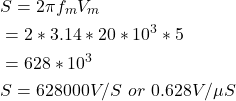## Applications of Slew Rate

Some of the applications of the Slew Rate include:

• In musical instruments, slew circuitry is used to provide a slide from one note to another i.e. portamento (also called glide or lag).
• Slew circuitry is used where the control voltage is slowly transitioned to different values over a period of time.
• In certain electronics applications where speed is required and the output needs to change over a period of time, software-generated slew functions or slew circuitry are used.

Want To Learn Faster? 🎓
Get electrical articles delivered to your inbox every week.
No credit card required—it’s 100% free.